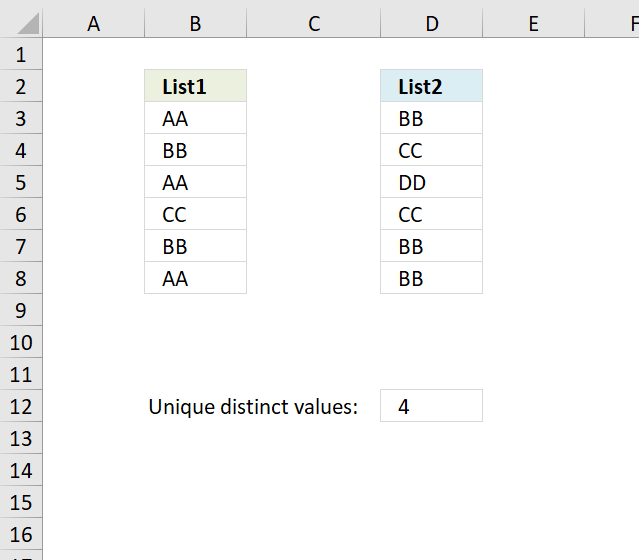Author: Oscar Cronquist Article last updated on November 19, 2018Formula in C12:

=SUM(1/COUNTIF(\$B\$3:\$B\$8, \$B\$3:\$B\$8))+SUM(IF(COUNTIF(\$B\$3:\$B\$8, \$D\$3:\$D\$8)=0, 1/COUNTIF(\$D\$3:\$D\$8, \$D\$3:\$D\$8), 0))

How to create an array formula

1. Double press with left mouse button on cell C12
2. Paste above formula
3. Press and hold Ctrl + Shift
4. Press Enter

Explaining formula in cell C12

Step 1 - Count values in cell range B3:B8

The COUNTIF function counts values equal to a condition or criteria.

COUNTIF(\$B\$3:\$B\$8, \$B\$3:\$B\$8)

becomes

COUNTIF({"AA";"BB";"AA";"CC";"BB";"AA"}, {"AA";"BB";"AA";"CC";"BB";"AA"})

and returns

{3;2;3;1;2;3}

Step 2 - Divide 1 with array

1/COUNTIF(\$B\$3:\$B\$8, \$B\$3:\$B\$8)

becomes

1/{3;2;3;1;2;3}

and returns

{0.333333333333333;0.5;0.333333333333333;1;0.5;0.333333333333333}

Step 3 - Sum values

The SUM function simply adds the numbers and returns the total.

SUM(1/COUNTIF(\$B\$3:\$B\$8, \$B\$3:\$B\$8))

becomes

SUM({0.333333333333333;0.5;0.333333333333333;1;0.5;0.333333333333333})

and returns 3.

Step 4 - Which values exist in cell range \$D\$3:\$D\$8

COUNTIF(\$B\$3:\$B\$8, \$D\$3:\$D\$8)=0

becomes

COUNTIF({"AA";"BB";"AA";"CC";"BB";"AA"}, {"BB";"CC";"DD";"CC";"BB";"BB"})=0

becomes

{2;1;0;1;2;2}=0

and returns

{FALSE; FALSE; TRUE; FALSE; FALSE; FALSE}

Step 5 - Convert TRUE to corresponding number

The IF function uses a logical expression (argument1) to determine which value to return (TRUE - argument2 , FALSE - argument3)

IF(COUNTIF(\$B\$3:\$B\$8, \$D\$3:\$D\$8)=0, 1/COUNTIF(\$D\$3:\$D\$8, \$D\$3:\$D\$8), 0)

becomes

IF({FALSE; FALSE; TRUE; FALSE; FALSE; FALSE}, 1/COUNTIF(\$D\$3:\$D\$8, \$D\$3:\$D\$8), 0)

becomes

IF({FALSE; FALSE; TRUE; FALSE; FALSE; FALSE}, {0.333333333333333;0.5;1;0.5;0.333333333333333;0.333333333333333}, 0)

and returns

{0;0;1;0;0;0}.

Step 6 - Sum array

SUM(IF(COUNTIF(\$B\$3:\$B\$8, \$D\$3:\$D\$8)=0, 1/COUNTIF(\$D\$3:\$D\$8, \$D\$3:\$D\$8), 0))

becomes

SUM({0;0;1;0;0;0})

and returns 1.

SUM(1/COUNTIF(\$B\$3:\$B\$8, \$B\$3:\$B\$8))+SUM(IF(COUNTIF(\$B\$3:\$B\$8, \$D\$3:\$D\$8)=0, 1/COUNTIF(\$D\$3:\$D\$8, \$D\$3:\$D\$8), 0))

becomes

3+SUM(IF(COUNTIF(\$B\$3:\$B\$8, \$D\$3:\$D\$8)=0, 1/COUNTIF(\$D\$3:\$D\$8, \$D\$3:\$D\$8), 0))

becomes

3+1

and returns 4 in cell D12.

Get Excel *.xlsx file

Count unique distinct values in two columns.xlsx